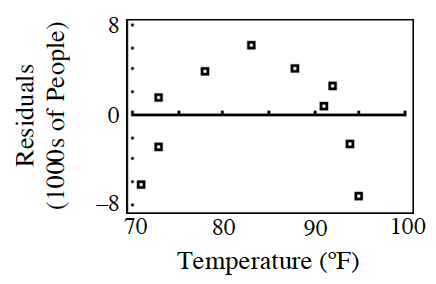### Home > CCAA8 > Chapter 6 Unit 7 > Lesson CCA: 6.2.3 > Problem6-86

6-86.

Marissa went with her friends to the amusement park on a beautiful spring day. The park was crowded. Marissa wondered if there was an association between the weather and attendance. From data she received at the theme park office, Marissa randomly picked ten Saturdays and analyzed the data.

1. Marissa calculated the least squares regression line $a = -14 + 0.41t,$ where a is the attendance (in $1000\text{s}$) and t is the high temperature ($º\text{F}$) that day. Interpret the slope in this context.

With each additional degree of temperature, we predict an increase of $410$ park visitors.
Make sure you know why this is the answer.

1. The residual plot Marissa created is shown at right. On days when temperatures were in the $80\text{s}$, would you expect the predictions made by Marissa’s model to be too high, too low, or pretty accurate?

What does it mean when the residuals are positive?
Are the actual values greater than or less than the predicted values?1. What was the actual attendance on the day when the temperature was $95º\text{F}$?

Substitute $95$ for t in the LSRL equation in part (a) to find what the LSRL predicts.
Then, use the equation actual − prediction = residual to find the actual value

2. Marissa drew the upper boundary line at $a = -7 + 0.41t$ and the lower boundary line at $a = -21 + 0.41t$. What are the upper and lower bounds for the predicted number of people attending when the temperature is $80º\text{F}$?

The predicted attendance is between 11,800 and 25,800 people. Show your work.

3. Would you rely on this model to make predictions? Why or why not?

Look at the residual plot. Is there a pattern? Note that the range for the predicted attendance in part (d) is very large, is this useful or not?